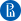up

# Probability Theory, Statistics and Exploratory Data Analysis• English

course language

• 6 weeks

course duration

• от 3 до 6 часов в неделю

needed to educate

• 3 credit points

This online course introduces you to the necessary sections of probability theory and statistics, guiding you from the very basics all way up to the level required for jump starting your ascent in Data Science.

The core concept of the course is random variable — i.e. variable whose values are determined by random experiment. Random variables are used as a model for data generation processes we want to study. Properties of the data are deeply linked to the corresponding properties of random variables, such as expected value, variance and correlations. Dependencies between random variables are crucial factor that allows us to predict unknown quantities based on known values, which forms the basis of supervised machine learning. We begin with the notion of independent events and conditional probability, then introduce two main classes of random variables: discrete and continuous and study their properties. Finally, we learn different types of data and their connection with random variables.

While introducing you to the theory, we'll pay special attention to practical aspects for working with probabilities, sampling, data analysis, and data visualization in Python.

This Course is part of HSE University Master of Data Science degree program.

## Format

The course takes place on the platform of the Higher School of Economics.

The course consists of 6 weeks. Each week contains video lectures, tests and materials for independent study. At the end of the course the pratical project awaits you.

## Course program

1. Conditional probability and Independence

During this week we discuss conditional probability and independence of events. Sometimes we can use this definition to find probabilities. Sometimes we check that this definition fulfills to assure whether events are independent. We discuss important law of total probability, which allows us to find probability of some event when we know its conditional probabilities provided some hypotheses and probabilities of the hypotheses. We also discuss Bayes's rule which allows us to find probability of hypothesis provided that some event occurred. We demonstrate how Python can be used for calculating conditional probabilities and checking independence of events.

2. Random variables

Random variable denotes a value that depends on the result of some random experiment. Some natural examples of random variables come from gambling and lotteries. There are two main classes of random variables that we will consider in this course. This week we'll learn discrete random variables that take finite or countable number of values. Discrete random variables can be described by their distribution. We'll consider various discrete distributions, introduce notions of expected value and variance and learn to generate and visualize discrete random variables with Python.

3. Systems of random variables; properties of expectation and variance, covariance and correlation.

Several random variables associated with the same random experiment constitute a system of random variables. To describe system of discrete random variables one can use joint distribution, which takes into account all possible combinations of values that random variables may take. We'll find some joint distributions, research their properties and introduce independence of random variables. Then we'll discuss properties of expected value and variance with respect to arithmetic operations and introduce measures of independence between random variables.

4. Continuous random variables

This week we'll study continuous random variables that constitute important data type in statistics and data analysis. For continuous random variables we'll define probability density function (PDF) and cumulative distribution function (CDF), see how they are linked and how sampling from random variable may be used to approximate its PDF. We'll introduce expected value, variance, covariance and correlation for continuous random variables and discuss their properties. Finally, we'll use Python to generate independent and correlated continuous random variables.

5. From random variables to statistical data. Data summarization and descriptive statistics.

This week we'll introduce types of statistical data and discuss models that are used to pass from statistical data to random variables. We'll introduce descriptive statistics of sample data, such as various measures of central tendency and statistical dispersion, and find correspondences between properties of random variables (population) and the sample descriptive statistics, which are essential for statistical predictions. We’ll talk about visualization of statistical data and learn to work with them in Python.

6. Correlations and visualizations

This week we’ll consider correlation in statistical data and find out how its' related to the level of dependance within the data and what it means for scatter plots. We’ll consider several types of correlation suitable for different types of data and discuss difference between correlation and causation. Finally, we’ll learn to visualize dependence between numeric variables and calculate correlation with Python.### Щуров Илья Валерьевич

Кандидат физико-математических наук
Position: Доцент кафедры высшей математики

## Programs, which includes this course

New ProgramStart date will be announced laterНИУ ВШЭ